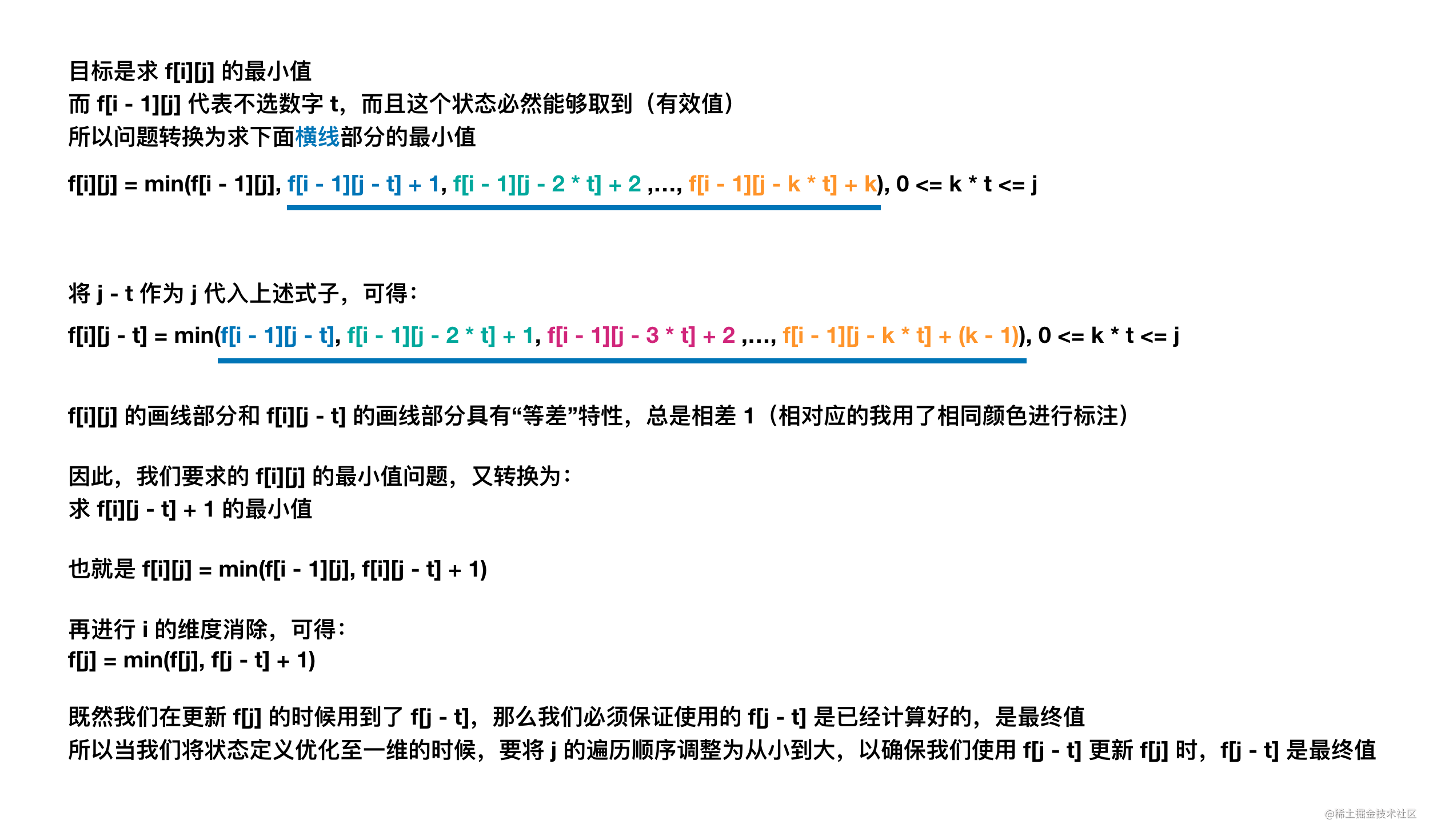# 【背包问题の第五讲】强化利用「等差」特性推导「完全背包」的核心要素

## 题目描述

「完全平方数」是一个整数，其值等于另一个整数的平方；换句话说，其值等于一个整数自乘的积。

输入：n = 12

输入：n = 13

• 1 <= n <= $10^4$

## 完全背包（朴素解法）

• 第一维 $i$ 代表物品编号

• 第二维 $j$ 代表容量

$f[i][j]$ 为考虑前 $i$ 个数字，凑出数字总和 $j$ 所需要用到的最少数字数量。

• 选 0 个数字 $i$，此时有 $f[i][j] = f[i - 1][j]$

• 选 1 个数字 $i$，此时有 $f[i][j] = f[i - 1][j - t] + 1$

• 选 2 个数字 $i$，此时有 $f[i][j] = f[i - 1][j - 2 * t] + 2$

...

• 选 k 个数字 $i$，此时有 $f[i][j] = f[i - 1][j - k * t] + k$

$f[i][j] = min(f[i-1][j-k*t]+k),0 \leqslant k * t \leqslant j$

class Solution {
int INF = -1;
public int numSquares(int n) {
// 预处理出所有可能用到的「完全平方数」
List<Integer> list = new ArrayList<>();
int idx = 1;
while (idx * idx <= n) {
idx++;
}

// f[i][j] 代表考虑前 i 个物品，凑出 j 所使用到的最小元素个数
int len = list.size();
int[][] f = new int[len][n + 1];

// 处理第一个数的情况
for (int j = 0; j <= n; j++) {
int t = list.get(0);
int k = j / t;
if (k * t == j) { // 只有容量为第一个数的整数倍的才能凑出
f[j] = k;
} else { // 其余则为无效值
f[j] = INF;
}
}

// 处理剩余数的情况
for (int i = 1; i < len; i++) {
int t = list.get(i);
for (int j = 0; j <= n; j++) {

// 对于不选第 i 个数的情况
f[i][j] = f[i - 1][j];

// 对于选 k 次第 i 个数的情况
for (int k = 1; k * t <= j; k++) {
// 能够选择 k 个 t 的前提是剩余的数字 j - k * t 也能被凑出
if (f[i - 1][j - k * t] != INF) {
f[i][j] = Math.min(f[i][j], f[i - 1][j - k * t] + k);
}
}

}
}
return f[len - 1][n];
}
}

• 时间复杂度：预处理出所有可能用到的数字复杂度为 $O(\sqrt{n})$，共有 $n * \sqrt{n}$ 个状态需要转移，每个状态转移最多遍历 $n$ 次，因此转移完所有状态复杂度为 $O(n^2 * \sqrt{n})$。整体复杂度为 $O(n^2 * \sqrt{n})$
• 空间复杂度：$O(n * \sqrt{n})$

## 完全背包（进阶）$f[j] = min(f[j], f[j - t] + 1)$

class Solution {
int INF = -1;
public int numSquares(int n) {
// 预处理出所有可能用到的「完全平方数」
List<Integer> list = new ArrayList<>();
int idx = 1;
while (idx * idx <= n) {
idx++;
}

// f[j] 代表考虑到当前物品为止，凑出 j 所使用到的最小元素个数
int len = list.size();
int[] f = new int[n + 1];

// 处理第一个数的情况
for (int j = 0; j <= n; j++) {
int t = list.get(0);
int k = j / t;
if (k * t == j) { // 只有容量为第一个数的整数倍的才能凑出
f[j] = k;
} else { // 其余则为无效值
f[j] = INF;
}
}

// 处理剩余数的情况
for (int i = 1; i < len; i++) {
int t = list.get(i);
for (int j = t; j <= n; j++) {
// 当不更新 f[j] 的时候，对应了二维表示中的 f[i - 1][j]

// 可以更新 f[j] 的前提是：剩余的 j - k * t 也能够被凑出
// 更新 f[j] 所依赖的 f[j - t] 对应了二维表示中的 f[i - 1][j - k * t]
if (f[j - t] != INF) f[j] = Math.min(f[j], f[j - t] + 1);
}
}

return f[n];
}
}

• 时间复杂度：预处理出所有可能用到的数字复杂度为 $O(\sqrt{n})$，共有 $n * \sqrt{n}$ 个状态需要转移，复杂度为 $O(n * \sqrt{n})$。整体复杂度为 $O(n * \sqrt{n})$
• 空间复杂度：$O(n)$

## 背包问题（目录）

1. 01背包 : 背包问题 第一讲

1. 【练习】01背包 : 背包问题 第二讲

2. 【学习&练习】01背包 : 背包问题 第三讲

2. 完全背包 : 背包问题 第四讲

1. 【练习】完全背包 : 本篇

2. 【练习】完全背包 : 背包问题 第六讲

3. 【练习】完全背包 : 背包问题 第七讲

3. 多重背包 : 背包问题 第八讲

4. 多重背包（优化篇）

1. 【上】多重背包（优化篇）: 背包问题 第九讲

2. 【下】多重背包（优化篇）: 背包问题 第十讲

5. 混合背包 : 背包问题 第十一讲

6. 分组背包 : 背包问题 第十二讲

1. 【练习】分组背包 : 背包问题 第十三讲
7. 多维背包

1. 【练习】多维背包 : 背包问题 第十四讲

2. 【练习】多维背包 : 背包问题 第十五讲

8. 树形背包 : 背包问题 第十六讲

1. 【练习篇】树形背包

2. 【练习篇】树形背包

9. 背包求方案数

1. 【练习】背包求方案数
10. 背包求具体方案

1. 【练习】背包求具体方案
11. 泛化背包

1. 【练习】泛化背包